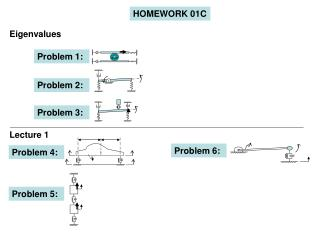DownloadDownload PresentationHOMEWORK 01C

# HOMEWORK 01C

Download Presentation## HOMEWORK 01C

- - - - - - - - - - - - - - - - - - - - - - - - - - - E N D - - - - - - - - - - - - - - - - - - - - - - - - - - -
##### Presentation Transcript

1. HOMEWORK 01C Eigenvalues Problem 1: Problem 2: Problem 3: Lecture 1 Problem 6: Problem 4: Problem 5:

2. Problem 1: The solution of Problem 1 in Homework 01B gives the equation motion below, Here, f(t) is the input, x(t) is the generalized coordinate. Find the eigenvalues of the system for the values of m=2 kg, k=3240 N/m ve c=380 Ns/m. Is the system stable? Write the form of free vibration response. Determine the values of Δt ve t∞ for the system’s response to be analyzed. Click for answers. Eigenvalue equation: 2.5ms2+2cs+2k=0 System is stable, form of the response: Δt=0.0022, t∞=0.7

3. Problem 2: The solution of Problem 2 in Homework 01B gives the equation motion below, Here, T(t) is the input, θ(t) is the generalized coordinate. Find the eigenvalues of the system for the values of m=1.8 kg, L=0.42m, k=32000 N/m, c=486 Ns/m. Is the system stable? Find undamped natural frequency and damping ratio. Determine the values of Δt ve t∞ for the system’s response to be analyzed. Click for answers. f0=63.6943 Hz, ξ=0.3375, Δt=0.7854 x 10-3 s, t∞=0.0465 s

4. Problem 3: The solution of Problem 3 in Homework 01B gives the equation motion below. Here, f(t) ve x1(t)are the inputs, xA(t) ve θ(t)are the generalized coordinates. Find the eigenvalues of the system for the values of m=20 kg, L=0.6 m, k=42000 N/m, c=2000 Ns/m. Is the system stable? Write the form of free vibration response. Find undamped natural frequency in terms of Hz and damping ratio. Determine the values of Δt ve t∞ for the system’s response to be analyzed. Click for answers. f0=4.33 Hz, ξ=0.648, Δt=0.0041 s, t∞=0.36

5. L2 L1 y2 y1 G yA yB c k c k m,I Problem 4: m=1050 kg, I=670 kg-m2 k=35300 N/m, c=2000 Ns/m L1=1.7 m, L2=1.4 m For the system shown in the figure, yA are yB the inputs, y1 and y2 are the generalized coordinates. Find undamped natural frequencies in terms of Hz and damping ratio of the system. Determine the values of Δt ve t∞ for the system’s response to be analyzed. Click for answers. f1=1.2968 Hz, f2=2.5483 Hz s1,2=1.8809±7.9283i (ξ=0.2308), s3,4=-7.2627±14.2698i (ξ=0.4536) Δt=0.0196 s, t∞=3.34 s Solution:

6. L2 L1 y2 y1 G yA yB c k c k m,I Solution: m=1050 kg, I=670 kg-m2 k=35300 N/m, c=2000 Ns/m L1=1.7 m, L2=1.4 m

7. k3 c3 f2 x2 m2 k2 c2 f1 x1 m1 k1 c1 Problem 5: m1=5.8 kg, m2=3.2 kg, k1=4325 N/m, k2=3850 N/m, k3=3500 N/m, c1=37.2 Ns/m, c2=33.5 Ns/m, c3=32 Ns/m. In the system shown in the figure, f1 and f2 are the inputs, x1 and x2 are the generalized coordinates. Find undamped natural frequencies in terms of Hz and damping ratio of the sytem. Determine the values of Δt ve t∞ for the system’s response to be analyzed. Click for answers. f1=4.6549 Hz, f2=8.4979 Hz s1,2=-3.76±29.01i (ξ=0.129), s3,4=-12.57±51.89i (ξ=0.235) Δt=0.0059 s, t∞=1.67 s Solution:

8. m1, L1 m2 θ k c yA Problem 6: m1=250 kg, m2=350 kg, k=37000 N/m, c=1500 Ns/m, L1=1.2 m In the system shown in the figure, yAis the input,θ(t)is the generalized coordinate. Find undamped natural frequencies in terms of Hz and damping ratio of the system. Click for answers. f=1.4692 Hz, s1,2=-1.7308 ± 9.0768i (ξ=0.1872). Solution: